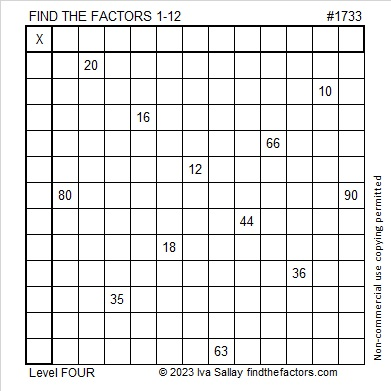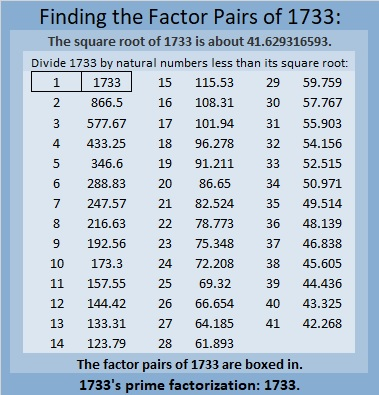# 1733 and Its Prime Number Substrings

Contents

### Today’s Puzzle:

Use logic to write each number from 1 to 12 in both the first column and the top row so that those numbers are the factors of the given clues.### Factors of 1733:

• 1733 is a prime number.
• Prime factorization: 1733 is prime.
• 1733 has no exponents greater than 1 in its prime factorization, so √1733 cannot be simplified.
• The exponent in the prime factorization is 1. Adding one to that exponent we get (1 + 1) = 2. Therefore 1733 has exactly 2 factors.
• The factors of 1733 are outlined with their factor pair partners in the graphic below.How do we know that 1733 is a prime number? If 1733 were not a prime number, then it would be divisible by at least one prime number less than or equal to √1733. Since 1733 cannot be divided evenly by 2, 3, 5, 7, 11, 13, 17, 19, 23, 29, 31, 37, or 41, we know that 1733 is a prime number.

### More About the Number 1733:

1733 is the sum of two squares:
38² + 17² = 1733.

1733 is the hypotenuse of a Pythagorean triple:
1155-1292-1733 calculated from 38² – 17², 2(38)(17), 38² + 17².

Here’s another way we know that 1733 is a prime number: Since its last two digits divided by 4 leave a remainder of 1, and 38² + 17² = 1733 with 38 and 17 having no common prime factors, 1733 will be prime unless it is divisible by a prime number Pythagorean triple hypotenuse less than or equal to √1733. Since 1733 is not divisible by 5, 13, 17, 29, 37, or 41, we know that 1733 is a prime number.

1733 is also the difference of two squares:
867² – 866² = 1733.
That means 1733 is also the short leg of the Pythagorean triple calculated from
867² – 866², 2(867)(866), 867² + 866².

1733 is a palindrome in three bases:
It’s 2101012 in base 3 because
2(3⁶) +1(3⁵) + 0(3⁴) + 1(3³) + 0(3²) +1(3¹) + 2(3⁰) = 1733,
It’s 565 in base 18 because 5(18²) + 6(18) + 5(1) = 1733, and
it’s 4F4 in base 19 because 4(19²) + 15(19) + 4(1) = 1733.

The next fact I learned from Twitter:

What prime numbers can be made with the digits of 1733?
3, 7, 13, 17, 31, 37, 71, 73, 137, 173, 313, 317, 331, 337, 373, 733, 1733.

The numbers in red are the 6 prime numbers that are substrings of 1733. I made a gif showing those 6 primes:make science GIFs like this at MakeaGif
1733 is quite a fascinating number!

This site uses Akismet to reduce spam. Learn how your comment data is processed.